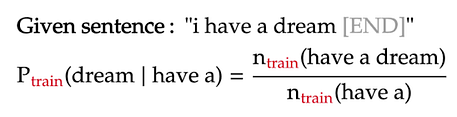# N-gram language models -Part2

## Background

In part 1 of my project, I built a unigram language model: it estimates the probability of each word in a text simply based on the fraction of times the word appears in that text.

The text used to train the unigram model is the book “A Game of Thrones” by George R. R. Martin (called train). The texts on which the model is evaluated are “A Clash of Kings” by the same author (called dev1), and “Gone with the Wind” — a book from a completely different author, genre, and time (called dev2).

In this part of the project, I will build higher n-gram models, from bigram (n=2) all the way to 5-gram (n=5). These models are different from the unigram model in part 1, as the context of earlier words is taken into account when estimating the probability of a word.

## Training the model

For a given n-gram model:

The example below shows the how to calculate the probability of a word in a trigram model:For simplicity, all words are lower-cased in the language model, and punctuations are ignored. The presence of the [END] tokens are explained in part 1.

## Dealing with words near the start of sentence

In higher n-gram language models, the words near the start of each sentence will not have a long enough context to apply the formula above. To make the formula consistent for those cases, we will pad these n-grams with sentence-starting symbols [S]. Below are two such examples under the trigram model:

From the above formulas, we see that the n-grams containing the starting symbols are just like any other n-gram. The only difference is that we count them only when they are at the start of a sentence. Lastly, the count of n-grams containing only [S] symbols is naturally the number of sentences in our training text: